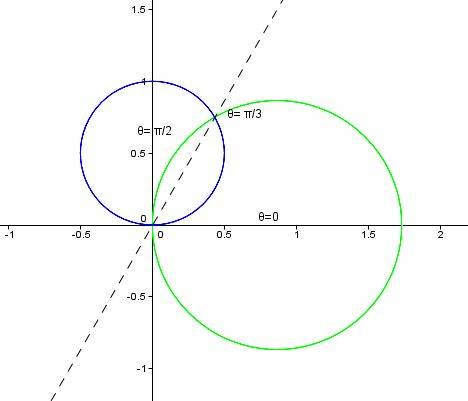# Find the area delimited by two polar curves

Astrowolf_13
Homework Statement:
Find the area limited by polar curves ##r=sin(\theta)## and ##r=\sqrt 3*cos(\theta)##.
Relevant Equations:
##r=sqrt(3)*cos(\theta)##, ##r=sin(\theta)##, ##0 \leq \theta \leq pi/2##.
I attempted to solve this problem by finding the angles of an intersection point by equalling both ##r=sin(\theta)## and ##r=\sqrt 3*cos(\theta)##. The angle of the first intersection point is pi/3. The second intersection point is, obviously, at the pole point (if theta=0 for the sine curve and theta=pi/2 for the cosine curve). I then attempted to find the required area by summing its "parts":
$$Area=0.5*\int_0^\frac{\pi}{3} (sin(\theta))^2\, d(\theta) + 0.5*\int_\frac{\pi}{3}^\frac{\pi}{2} (\sqrt 3 *cos(\theta))^2\, d(\theta)$$
The final area I got is ##5*\pi/24 + \sqrt 3 /4##, which I checked on the Internet. However, my teacher disregarded this solution as incorrect (because, as I quote, line at angle pi/3 is not a boundary of either ##r=sin(\theta)## or ##r=\sqrt 3 *cos(\theta)##) and asked to use a different method to find the area, and this is what I'm struggling to do.
So the question is: is there any other method of finding this area or did I get the description of the homework statement wrong?
The graph should look like this:Last edited:
•etotheipi

Mentor

You can solve this without integrals, however. Let O be the origin and P the other intersection of the circles. Let M be the center of the cosine circle. Draw this triangle, then find the angle phi at M. From there you can calculate the "left" part of your area with simple geometry. Do the same for the other circle.

It's also possible to convert the circles to Cartesian coordinates and integrate there, but why would someone want to do that.

••etotheipi and member 587159
•